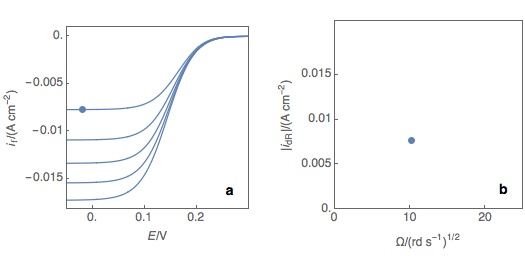Topic 10 min read# Levich analysis: the principles

Latest updated: August 9, 2023

Determination of the diffusion coefficient for a redox reaction

The Levich experiment consists of performing a series of voltammetries on a Rotating Disk Electrode at various rotation speeds. It is important to use a potential scan rate small enough so that the reaction occurs reversibly, ie the forward and backward curves are superimposed. The rotation speeds should also be small enough that the reaction can be considered reversible, usually, 500 to 5000 RPM are correct values.

All voltammograms should show, from a certain potential value, a current plateau (Fig. 1a). The value of this mass-transport-limited current depends on the square root of the rotation speed following the Levich equation .Figure 1: (a) Voltamogramms resulting from the Levich experiment. The dots are the {I,E} points
used to plot the Levich line on the right. (b) Levich plot with the linear regression allowing the
determination of the Levich slope and the diffusion coefficient of the reduced species O.

If we plot the plateau reduction current $|i_\mathrm{dR}|$ as a function of the square root of the rotation speed (Fig. 1b), we can obtain the Levich line (Fig. 1c), whose slope can be used to determine the diffusion coefficient of the involved redox species, given the number electrons in the reaction and the bulk concentration of the redox species.

The Levich slope $p_\mathrm{Lc}$ writes for a species O being reduced for a rotation speed expressed in $\mathrm{rad\,s^{-1}}$:

$$p_\mathrm{Lc}=-0.620\,n\,F\,O^\mathrm{bulk}\,D\mathrm {_O}^{2/3}\,{\nu}^{-1/6}$$

With $F$ the Faraday constant, $O^\mathrm{bulk}$ the bulk concentration of the species O in  $\mathrm{mol\,cm^{-3}}$, $D\mathrm {_O}$ the diffusion coefficients of O in $\mathrm{cm^2\,s^{-1}}$, ${\nu}$ the cinematic viscosity in $\mathrm{cm^2\,s^{-1}}$.

Note that if the diffusion coefficient and the concentrations are known, the number of electrons can be determined using the Levich slope.

The Levich analysis tool is part of EC-Lab® software and is described in more detail in application note 56  as well as the dedicated YouTube video .

Note that using the Koutecký-Levich analysis tool, you can derive the standard kinetic constant of the redox reaction. This analysis is also available in EC-Lab® and is explained in more details in the application note 56 as well as the in the corresponding topic  and YouTube video .

 Application Note 56: Measurement of electrochemical reaction kinetics: The Levich and Koutecký-Levich analysis tools

 YouTube video, BioLogic Video Channel: The Levich plot and analysis in EC-Lab®

 YouTube video: The Koutecký-Levich plot and analysis in EC-Lab®

RDE Rotating disk electrode BluRev BluRev RDE Levich steady-state analysis

## Related products

Work smarter. Not harder.

Tech-tips, theory, latest functionality, new products & more.

No thanks!